megaCalculator Information Page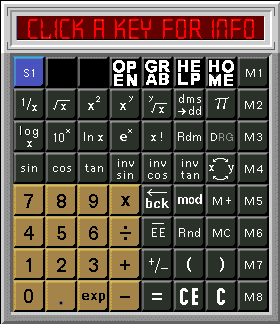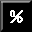The Percent key can be found by clicking S1 until you toggle through to the S3 bank of special keys. Percent Calculates the percentage of a quantity. e.g. 100 - 9% returns 91 100 + 9% returns 109 Open Opens a separate window to allow the user to enter digits in a text field. Once the user enters the number, they must then press Grab to transfer it to the calculator. Grab Grabs the value from the separate opened window. Will allow you to perform statistical calculations and pull the value into the calculator. Help Opens the help window. Home Opens the calculator home page. digits 1 thru 0 and '.' 1 through 0 and the decimal point '.' are used to create the value of the entered number. Enter them by clicking on the keys with your mouse, just as using a hand-held calculator. operators +, -, x, ÷, = +, -, x, ÷, = are used as operators. They function just as in math equations. When you try to divide a number by 0, you will get an error message. You must then re-enter a proper number. mod The symbol for Mod is often represented as % but Mod should not be confused with the common percent function. This operator is like the hands of a clock. 13:00 hours in Military time is the same as 1:00 on a standard clock face. This is equivalent to 13 mod 12. You can think of A_mod_B as the remainder of the equation A divided by B. exp The exp key is the way to enter an exponent into a number. Enter the digits with a decimal point(if any) and then press "exp." Then enter the exponent. If it is a negative exponent, press "+/-". For example, enter 5555.33 then press "exp", then enter 3 and then "+/_". When you then press "=" you will get 5.55533, which is what you entered.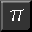pi The pi key is the way to enter the constant pi which is approximately 3.14159265. Rnd (round) This function returns the display value rounded to an integer. 12.5 is rounded to 13. 12.49999 is rounded to 12.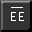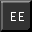Not EE, EE This changes the display from standard mode to exponential mode and back. Enter a standard number, press "EE", then press "=". The display will change to exponential mode. C, CE "C" clears the calculator, except for memories, and resets all flags to default. "CE" clears the entry, but leaves the various settings alone. Several types of operations do not permit the user to enter a new number unless they press "=" or "CE" or "C". M1 thru M8 These are 8 memory registers. Pressing each, recalls the value currently stored in that memory. M+, MC Pressing "M+" and one of the memory registers adds the display value to that register. Pressing "MC" and one of the memory registers clears that register. () The parentheses allow you to group parts of an equation just like a standard calculator. For example: (6x(1+7)+((3x4)ln-1)x5)=55.424533 +/- This key changes the sign of the entered value. If entered while entering an exponent this makes the the exponent negative. Bck (backspace) If pressed while entering a number, causes the entry to backspace.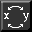(x exchange y), x<>y Causes the displayed value, the 'x' register, to exchange with a hidden 'y' register. This is required for several functions to work. sin, cos, tan, inv sin, inv cos, inv tan, DRG The common trigonometry functions. The DRG key cycles to display the proper angle entry unit. D for degrees, R for radians, and G for gradients. Be aware, the tan of 90 degrees or 100 grads is actually infinity, but due to round off error the calculator avoids returning an error. log x, 10x, ln x, ex Common log, xth magnitude, natural log, and e to the xth.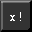x ! x factorial or 1x2x3x4x….(X-1)xX Rdm Returns a random number less than or equal to the display value. Always resolves to zero.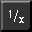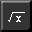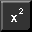1/x, sq root of x, x2 Reciprocal, square root, square of display.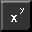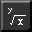xY, Yroot of x Display to the power of the 'y' register and display to the root of the 'y' register. You must enter a value into the 'y' register first to use these two functions. For example: Enter 20, press x<>y, enter 3, press x Y, yields 3486784401 dms >dd Degrees, minutes, seconds to decimal degrees. Enter dms as six or seven digits, the last two being seconds, the next two being minutes, and the first two or three being degrees. S1 thru S3. . . This button reassigns 18 special function keys to new functions. Keys in rows two, three, and four, columns one through six can be redefined by pressing this shift button. Repeated clicks will toggle through the available functions 18 special keys at a time. Not all keys in every bank of 18 are defined so keep an eye on this feature for continuing development. ©1996-97 Don Benish ~ Benish Industries